# Power, efficiency, colinearity, and variance inflation

### Dependencies and setup

This walkthrough uses CANlab object-oriented analysis functions with SPM12 basic functions (e.g., image reading/writing, HRF) in Matlab.
Do you have the relevant tools on your Matlab path?
which create_design_single_event
If you get an error, you don't! You need the Canlab Core repository. See this page for setup instructions.
You also need the Statistics and Machine Learning Toolbox in Matlab. Try this to check:
if isempty(ver('stats')), warning('You do not have the statistics toolbox on your Matlab path. You may/will get errors'); end

### Experimental design

In previous labs, we constructed a single-subject fMRI design for multiple regressors (e.g., responses to famous faces upright and inverted), in a sparse event-related design.
We constructed a model (X), which is the predicted fMRI signal in a brain area that responds to faces, and fit it to the data. Fitting the model involves solving the equation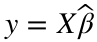, where y is a brain time series (a vector), Xis the model or "design matrix", and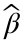is the unknown vector of regression slopes or "parameters".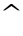or "hat" means something that is estimated from the data during model fitting. This model is fit to a single brain time series from a single person - for example, the average signal in a person's pre-identified "fusiform face area".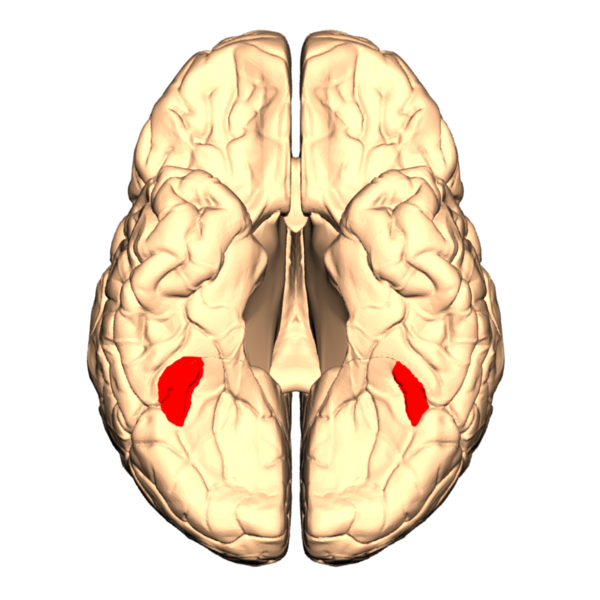### Create a simple, one-event design

TR = 2; % Repetition time for scans; one image every TR; in seconds
ISI = 18; % Inter-stimulus interval in sec (min time between events)
eventduration = 3; % Duration of 'neural' events in sec
HPlength = 128; % High-pass filter length in sec
dononlin = 0; % Nonlinear saturation model (0 = no, 1 = yes)
create_figure('design');
[X, e] = create_design_single_event(TR, ISI, eventduration, HPlength, dononlin);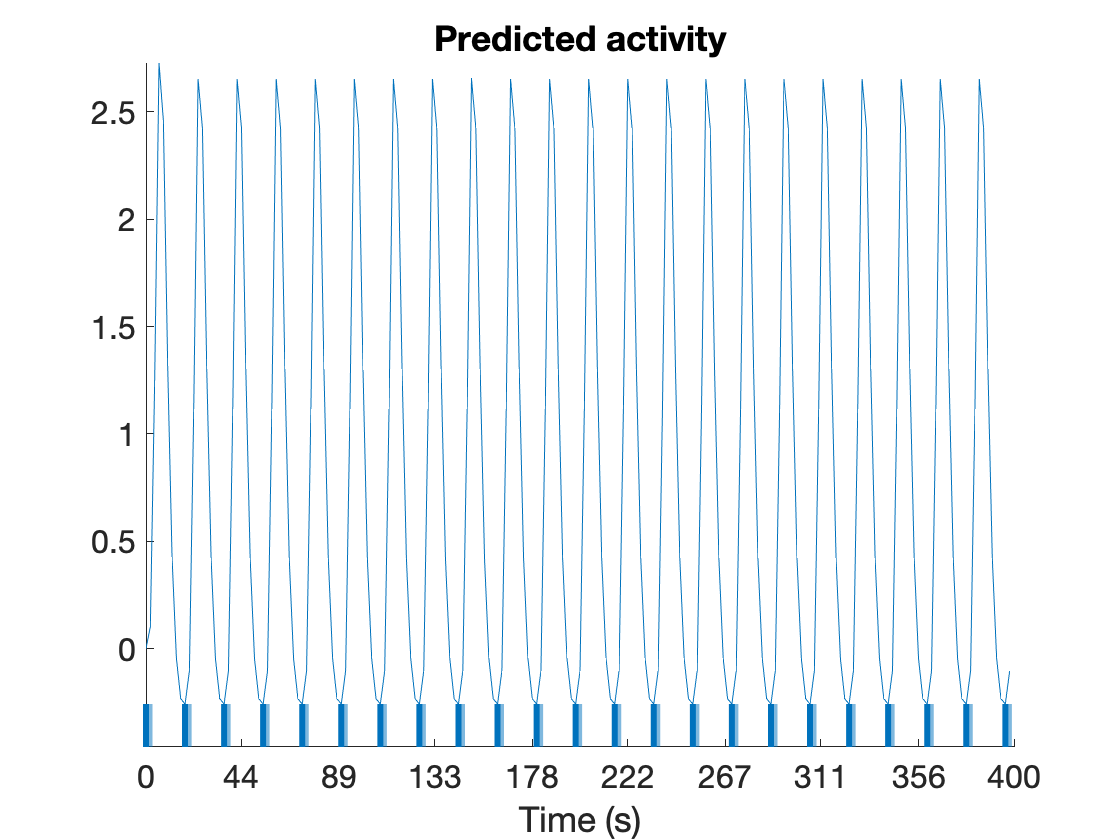drawnow, snapnow

### True and false positives, effect size, and power

An important consideration for any fMRI design is its power. Statistical power is the ability to detect a true response should one exist (i.e., obtain a significantfor any given regressor). Power is defined as the "hit rate" for a statistical test, or the probability of finding a significant result.
Power depends on the true effect size present in the data, and also on the acceptable false positive rate. In addition, different statistical tests can be more or less powerful at detecting the same true effect. Also, different fMRI designs can be more or less powerful depending on how the stimuli are arranged, which affects how they interact with the hemodynamic response (HRF) and scanner noise.
We'll go over a (very!) brief primer on some basic concepts. First, we have to set an acceptable false positive rate, the chances of calling an effect significant in the absence of one (Ho is true). If you never want to detect a false positive, you shouldn't call anything significant ever, no matter how strong the evidence! But in practice, we want to balance false positives against the ability to find effects. The convention is to set the acceptable false positive rate to a fixed value, denoted α. This is where p < 0.05 comes from -- alpha is 0.05. Power is complementary, and it's denoted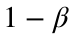(not to be confused with regression slope betas - sorry!), for reasons we'll see below.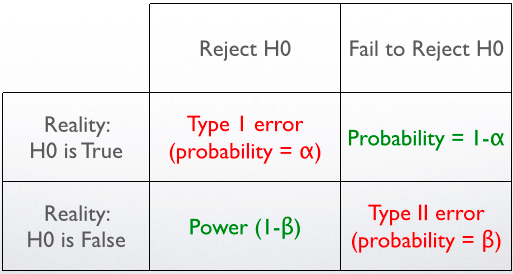Another way to view this is by thinking of two possible universes, one in which Ho is true, and the other in which Ho is false (H1 is true below). Given each of these two universes, the observed effect magnitude will vary because the data are noisy, and we can assume this variability follows a normal distribution. Therefore, these two universes are described by two Normal curves, as shown below. The decision point to reject the null hypothesis is shown as a location on the x-axis, which indexes effect magnitude. α is the proportion of cases where Ho is true but the effect magnitude is above threshold to reject, or "false alarms"/"false positives". This proportion is the area under the Ho curve, where all probability values sum to 1. They sum to 1 because in the universe where Ho is true, the probability that the effect falls between -infinity and +infinity is 1. β is the proportion of cases where Ho is false (H1 is true) and we fail to reject ("misses").is the area under the curve where H1 is true and we correctly reject the null, which is the power.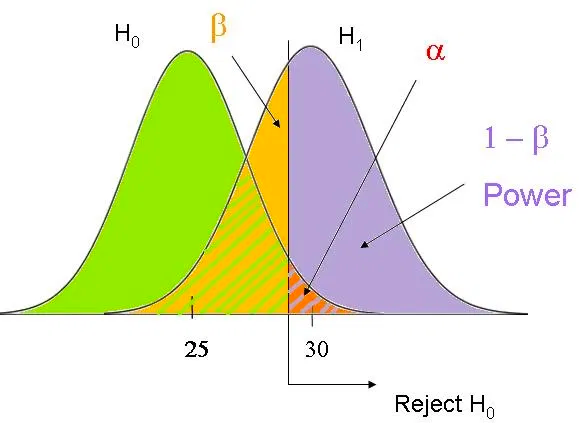Where is the effect size here? It's the distance between the peaks for H0 and H1. This reflects how much signal is there to detect, regardless of the statistical threshold for rejecting H0.
Effect sizes are measures of signal to noise ratio, i.e., effect magnitude / effect standard error. Thus, the larger the true signal relative to the noise, the higher the power.
To give us an intuition about power, we'll run a simulation. We'll generate 5,000 different datasets, with the same true effect but different random noise. The true effect magnitude will depend on the desired effect size. We'll standardize (z-score) the first column of X, the "true" signal (our "faces" regressor), and multiply it by the desired effect size. Then, we'll add noise, with a standard deviation fixed at 1 is fixed at 1, because y = X(:, 1) [] + noise in the code below. (Note that because we're generating autocorrelated noise, the observed standard deviation will be a bit larger due to the autocorrelation.)
You can set the effect size with the slider. Every time you adjust the slider, the simulation will run again. The observed power is calculated as the percentage of samples in which we obtain a significant result.
% Set the noise level (formally, noise standard deviation) here:
% -------------------------------------------------------
effect_size =0.5
effect_size = 0.5000
n_iterations = 5000; % Run this many iterations in our simulation
pval = Inf .* ones(n_iterations, 1); % Initalize P-value array for all sims
true_signal = effect_size .* zscore(X(:, 1)); % do this to standardize the effect magnitude
for i = 1:n_iterations
% Observed effect in sample
% -------------------------------------------------------
noise_ac = noise_arp(size(X, 1), [.7 .3]); % autocorrelated noise
y = true_signal + noise_ac; % simulated data
% Model fit
% -------------------------------------------------------
[b dev stats] = glmfit(X(:, 1), y);
pval(i, 1) = stats.p(2);
end
is_sig = pval < 0.05; % which are significant
detection_power = sum(is_sig) ./ length(is_sig);
fprintf('Power is %3.1f%%\n', 100 .* detection_power)
Power is 43.6%

Now let's do an exercise that will require a bit of coding. You can start with the code above, and add an outer loop that saves an estimate of power for a series of different true effect sizes. We'll operationalize effect size here as . Then, you'll make a graph of power vs. effect size.
This will start to introduce you to writing bits of code on your own. Try it on your own, and then check out the example code. If you get stuck, you can use bits of the example code, too -- but I made the font small so you can try it on your own first.
Before you start: What would you predict about the relationship between power and effect size? Will it be linear or nonlinear? What will the power be when the effect size is zero?
effect_sizes = [0:.1:1.2]; % here is a reasonable range of effect sizes and a way to define them
observed_power = zeros(size(effect_sizes)); % Create a placeholder array for power the same size as effect_sizes
for j = 1:length(effect_sizes)
true_signal = effect_sizes(j) .* zscore(X(:, 1)); % do this to standardize the effect magnitude
for i = 1:n_iterations
% noise_ac = noise_arp(size(X, 1), [.5 .1]); % autocorrelated noise
% noise_ac = randn(size(X, 1), 1); % Gaussian random noise, std=1
y = true_signal + noise_ac; % simulated data
% Model fit
% -------------------------------------------------------
[b dev stats] = glmfit(X(:, 1), y);
pval(i, 1) = stats.p(2);
end % inner loop, iteration
is_sig = pval < 0.05; % which are significant
observed_power(j) = sum(is_sig) ./ length(is_sig);
fprintf('Effect size %3.2f: Power is %3.1f%%\n', effect_sizes(j), 100 .* observed_power(j))
end % outer loop, j (effect size)
figure; plot(effect_sizes, observed_power)
xlabel('Effect size')
ylabel('Power')
1. Describe the relationship between effect size and power
2. What is the "power" when the effect size is zero? If there is no true effect, the chances of finding an effect should be the alpha level -- the acceptable false positive rate level. This is the P-value cutoff we chose (0.05). Why might it be higher? How could you verify (or explore) why it might be higher?
3. Re-run the simulation, but replace the autocorrelated noise with Gaussian (normally distributed, not autocorrelated) noise. The code below will help. What do you notice now about the power when the effect size is zero?
noise_ac = randn(size(X, 1), 1); % Gaussian random noise, std=1

### Design efficiency

e is the "efficiency", a relative metric of how good the design is compared with others with the same number of time points. We can use efficiency to explore which choices make a "better" design and which create problems. We'll explore this a bit here.
We won't go into a lot of detail here, but e is proportional to power. Efficiency, and therefore power, vary across designs as different choices are made -- e.g., do you scan for longer or shorter? How many event types (or conditions) are included? How much space is there between events?
Efficiency (e) the reciprocal of the design-related component of the standard error. Previously, we saw that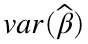is a measure of error variance, and it's square root is the standard error of the estimate, which is the denominator of the t statistic. So highermeans less power.
To estimate, we need to know how noisy the data is. The noise (or error) variance is usually denoted with σ, so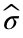is the estimated noise variance. Specifically,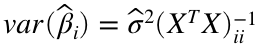, where i denotes the ith column of X (i.e., regressor), and ii denotes the ith diagonal of the matrix resulting from the multiplication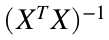. -1 here is the inverse operator.
But here, we don't have any data yet! So while we can't calculate, we can notice that part of the equation depends purely on the design matrix (X). So efficiency can be higher or lower independent of what the measurement error/noise level is. Efficiency is thus defined as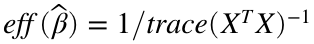, where the trace is the sum of diagonal elements.
For a more detailed explanation and general experimental design recommendations see this overview by Rik Henson, or this blog post on efficiency in experimental designs.
Let's explore this using our simple, one-event design:

#### Create a simple, one-event design

TR = 2; % Repetition time for scans; one image every TR; in seconds
ISI = 18; % Inter-stimulus interval in sec (min time between events)
eventduration = 3; % Duration of 'neural' events in sec
HPlength = 128; % High-pass filter length in sec
dononlin = 0; % Nonlinear saturation model (0 = no, 1 = yes)
create_figure('design');
[X, e] = create_design_single_event(TR, ISI, eventduration, HPlength, dononlin);
drawnow, snapnowfprintf('Efficiency: %3.2f\n', e)
Efficiency is e. More is better. Explore efficiency here by changing some of the parameters.

1. Make the events really brief (0.5 sec). What happens to the efficiency?
2. Make the events really long (17 sec). What happens to the efficiency?
3. What are some other ways you might be able to increase the efficiency of the design?
5. Explore different event durations systematically by running the code below. Make a plot of the curve showing efficiency from 1 to 18 sec event duration. Where is efficiency maximal? Why does this make sense?
create_figure('varying designs');
for dur = 1:18
[X, e(dur)] = create_design_single_event(TR, ISI, dur, HPlength, dononlin);
end
figure; plot(1:18, e)
xlabel('Event duration')
ylabel('Efficiency')
Block designs
Now, let's create a block design.
scanLength = 480;
nconditions = 5;
blockduration = 18;
create_figure('block design');
[X, e] = create_block_design(scanLength, TR, nconditions, blockduration, HPlength, dononlin);